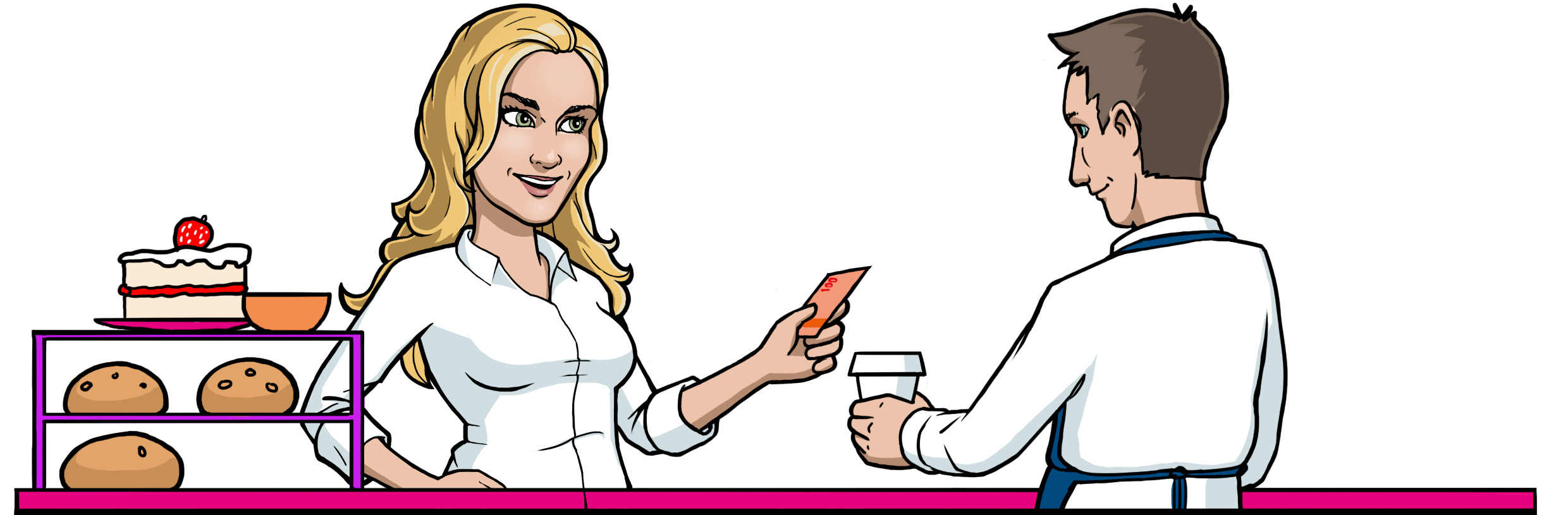Math Topics

# How Value-added Tax Is Calculated

In many countries around the world, prices on goods and services include a value added tax (shortened to VAT). This means that when you purchase an item in a store, a tax is calculated on the price of the item, and that amount goes straight to the government as a fee. There are some areas that are exempt from VAT, but they are very rare, so we won’t consider them here.

A common rate for VAT is $20$ %.

In the United States, there is no VAT, but there is a similar tax charged by many state and local governments called sales tax. You can use the same formula to calculate prices after sales tax as you use to calculate prices including VAT (see the Rule below), although the rate is much lower, usually around $7$ or $8$ %.

Now you’ll learn how to find the price of an item before and after VAT. Items that have VAT included in their price are denoted as price incl. VAT, and items that have VAT excluded, price excl. VAT.

Rule

### FindingthepriceincludingVAT

Example 1

An item costs \$$\text{}40\text{}$ excluding VAT. The VAT for this item is $\text{}20\text{}$%. How much is the item including VAT?

The calculation goes like this:

The price of this item incl. VAT is \$$48.00$.

Formula

### Findingthepriceexcl. VAT

Example 2

A grocery store chain has advertised that they have cut the VAT on fruits and vegetables down to $\text{}15\text{}$%. On the shelf in the store, the price is written incl. VAT, but it is removed at the register. The VAT for foods is $\text{}15\text{}$%. If you buy apples for $\text{}\text{}1\text{}$ including VAT, what will you eventually pay at the register?

You know that when you have a percentage of $15$, the growth factor will be $1.15$. We use this to find the price of the item without VAT.

The apples will cost \$$0.87$ without VAT.